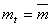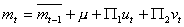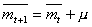# Prof. Bryan Caplan

bcaplan@gmu.edu

http://www.gmu.edu/departments/economics/bcaplan

Econ 816

Spring, 2000

HW#2

Part 1: Mathematical Problems

1.  Re-do the Lucas islands model presented in the notes, assuming that the money supply is constant; i.e.,.  Intuitively explain your results.

2.  For the Lucas islands model, solving the problem where monetary authorities target the nominal interest rate is particularly difficult.  You have to conjecture two equations:AND.

Why do these equations make sense?  Use basic monetary and RE principles to rationalize them.  You do not need to solve the problem.

3.  Romer, problem 9.4.

Part 2: Analytical Problems

TYPE succinct (1 page or less!) answers to each of the following:

1.  When do deviations from "rational expectations in the aggregate" occur?  When do severe instances of the "bias towards zero in aggregate perceptions" appear?  Is there any common pattern that both deviations from RE share?

2.  Agree or disagree: Caplan's "rational irrationality" analysis suggests that the rational expectations assumption probably applies in all macroeconomic models so long as they do not have a political component.

3.  Pick a specific government policy that you think is inefficient.  Which, if any, of Caplan's findings in "Systematically Biased Beliefs About Economics," would explain the existence of this policy?  Do Caplan's findings make "special interest"/rent-seeking explanations of your example superfluous?